# MCQs on Electric Circuits

##### Page 41 of 63. Go to page 1 2 3 4 5 6 7 8 9 10 11 12 13 14 15 16 17 18 19 20 21 22 23 24 25 26 27 28 29 30 31 32 33 34 35 36 37 38 39 40 41 42 43 44 45 46 47 48 49 50 51 52 53 54 55 56 57 58 59 60 61 62 63
01․ The phase angle difference between current and voltage is 90o, the power will be
minimum.
maximum.
zero.
VI.

The expression of active power P = VIcosθ . Where, V is voltage, I is current and θ is the angle between current and voltage. Here, this θ  = 90o. ∴ Power P = VIcos90o = 0. (Since, cos90o = 0)

02․ Kirchhoffs laws are valid for
linear circuit only.
passive time invariant circuits.
non-linear circuits only.
both linear and non-linear circuits.

Linear circuits obey Ohms law. Kirchhoffs laws are valid for those elements that obey Ohms law.

03․ For the circuit shown below the value of R is adjusted so as to make the current in RL equal to zero. Calculate the value of R.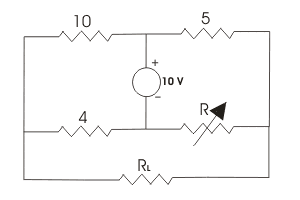1 Ω.
2 Ω.
3 Ω.
4 Ω.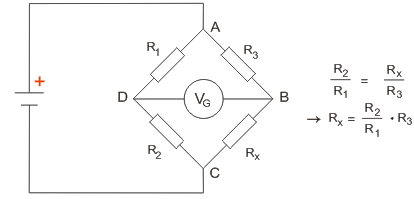As per Wheatstone bridge principle,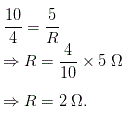04․ In the circuit shown in figure if I1 = 1.5 A, then I2 will be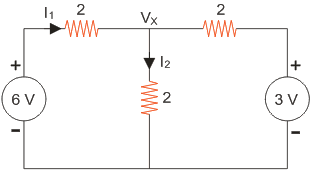2 A.
1.5 A.
1 A.
0.5 A.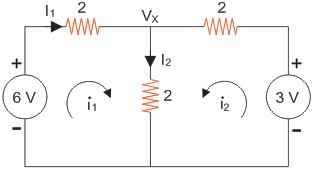Applying KVL in the loops, i1 = I1 =I2 and i2 = 0. So I2 = 1.5 A.

05․ In the circuit shown in the figure the voltage across the 2 Ω resistor is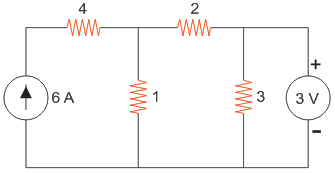1 V.
2 V.
3 V.
4 V.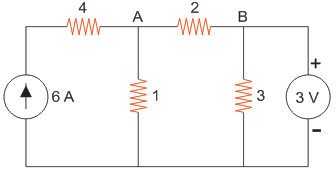VB = 3V Applying KCL at node A, VA = 5V Therefore, the voltage across 2 Ohm resistor is 5-3 = 2V.

06․ The value of current I flowing in the 1 Ω resistor in the circuit shown in the given figure will be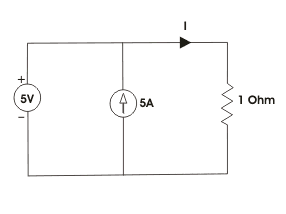5 A.
6 A.
0 A.
10 A.

5A current flows through the 1 Ohm resistor as no current flows to the voltage source.

07․ The voltage across the 1 Ω resistor between the nodes A and B of the network shown in the given figure is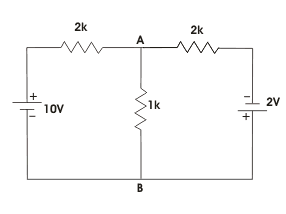1 V.
2 V.
3 V.
4 V.

Applying KCL at node A, VA = 2V.

08․ In the network shown, what is the current I in the direction shown?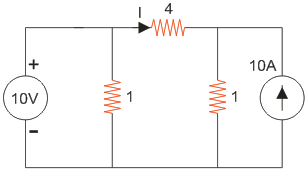0 A.
1 / 3 A.
5 / 6 A.
4 A.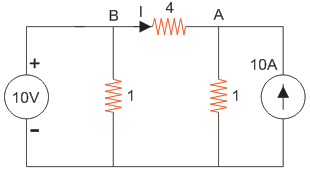VB = 10V. Applying KCL at node A, VA = 10V. Therefore, the current I is 0.

09․ An electrical circuit with 10 branches and 7 nodes will have
10 loop equations.
7 loop equations.
3 loop equations.
4 loop equations.

Number of loop equations = branches - nodes + 1 = b - n + 1 = 10 - 7 + 1 = 4 loop equations.

10․ In given figure, the value of resistance R in Ω is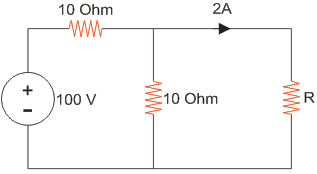10.
20.
30.
40.

The given circuit can be simplified by replacing voltage source by equivalent current source as below,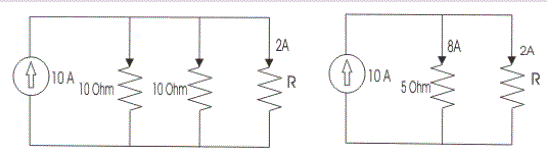The current through 5 Ω resistor is 8 A hence voltage across it is 8 X 5 = 40 V and this is the voltage across unknown resistor R. As current through R is 2 A.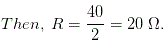<<<3940414243>>>